International Journal of Environmental Monitoring and Analysis
Volume 4, Issue 2, April 2016, Pages: 56-64

A Regression Model for Estimating Salinity in the South Eastern Mediterranean Sea

Maged Mohamed Abdel Moneim Hussein

Marine Physics Laboratory, Division of Marine Environment, National Institute of Oceanography and Fisheries, Alexandria, Egypt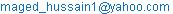Maged Mohamed Abdel Moneim Hussein. A Regression Model for Estimating Salinity in the South Eastern Mediterranean Sea. International Journal of Environmental Monitoring and Analysis. Vol. 4, No. 2, 2016, pp. 56-64. doi: 10.11648/j.ijema.20160402.13

Received: March 15, 2016; Accepted: March 24, 2016; Published: April 5, 2016

Abstract: This paper attends the problem of estimating salinity for a southeastern Mediterranean Sea. The main objective of the present study is the estimation of salinity profiles in the upper 500m from measurements of temperature profiles and surface salinity. 465 Temperature and salinity profiles were selected for this study, taken from expeditions carried out by research vessels Yakov Gakkov and Vladimir Parshin, of former Soviet Union during the period 1987-1990. The empirical relationship between salinity and temperature in southeastern Mediterranean Sea is quantified with the help of local regression. Differences in salinity's co-variability with temperature and with longitude, latitude and day of year from eastern to western part of the study area suggested that the region may be achieving more accurate salinity estimates. Eight methods were used for estimating salinity profiles in the present study. The results obtained from method 5 (Surface salinity added to fourth degree polynomial of temperature) were better than other methods for the upper 130m, while method 8 (longitude, latitude and day of year added to third degree polynomial of temperature) were better for the rest depths.

Keywords: Mediterranean Sea, Salinity, Temperature Profile, Regression

1. Introduction

Seawater temperature (T) measurements are much cheaper and easier to do than the measurements of salinity (S) consequently; the temperature values dataset is much bigger than the salinity values dataset. The implementation of multi-parametric data assimilation schemes in ocean forecasting models presuppose the use of factual salinity values estimated from temperature profiles. The logical way is based some physical assumptions and climatology datasets . There is no dynamical relationship between temperature and salinity, but temperature and salinity can present strong empirical relationships within the different water masses. So, there is a global need to estimate the salinity. The relationship between Salinity and temperature and other observables varies spatially and also depend on the region. So, the mission of developing capability for salinity estimation must be approached spatially region by region .

The salinity may be estimated from the measurements of previous temperature . This concept has often been accomplished using temperature and salinity climatological mean profiles : the estimate for salinity at observed value of temperature can be read from the temperature-salinity curve plotted from the climatological means.

Many authors have built upon Stommel's suggestion, e.g., [5,6,7,8,9]. The (T-S) relationship has been expressed by [10,11] through regression models: for any required depth, salinity estimate can be regressed on temperature and on many other convenient variables such as surface salinity, longitude, latitude, etc., which may be provide information about salinity.

Due to the weak correlation between the salinity and temperature in the near surface region, regressing salinity on temperature and Stommel's method were not expected to perform well for salinity estimation. So, we need other source of information, such as longitude, latitude, climatic indices, or day of the year, to be used as predictors of near surface salinity. While salinity value at surface proved to be quite useful in the upper 50m in different regions [10,11]. Linear regression can take advantage of high density vertical sampling to focus on the variability at definite depths, which can be chosen as required along the water column [10,12,13,2]. Moreover, regression models often can capture systematic spatial variability (local) by inclusive longitude and latitude in that models. Other approach proposed  to estimate the climatological salinity profiles in the ocean, is that employs empirical orthogonal function in combination with clustering technique to divide the world's ocean into climatological regions. A neural network model is also proposed  for reconstructing ocean salinity profiles from sea surface parameters only (latitude and sea surface salinity are the most relevant surface parameters in the prediction of salinity profiles).

The present paper aims to carry out suitable regression methods (models) to estimate salinity profiles in the upper 500m from temperature profiles measurements, sea surface salinity and other correlates of salinity in the southeastern Mediterranean Sea.

2. Material and Methods

2.1. Materials

Temperature and salinity profiles (465) are acquired by the Soviet Union research vessels  have been used in this study to carry out empirical relationships between salinity and temperature for the region from 22°E to 32°E and from 31°N to 37°N, (10°´6°) are shown in (Figure 1). These profiles have been collected during years 1987 to 1990 with the bulk of the data collected in spring season (March and April). The profiles had data listed in different intervals (0, 10, 20, 30, 40, 50, 60, 70, 80. 90, 100, 110, 120, 130, 140, 150, 160, 170, 180, 190, 200, 210, 220, 230, 240, 250, 260, 270, 280, 290, 300, 310, 320, 330, 340, 350, 360, 370, 380, 390, 400, 425, 450, 475 and 500m). About 40 profiles only reach to 400m depth.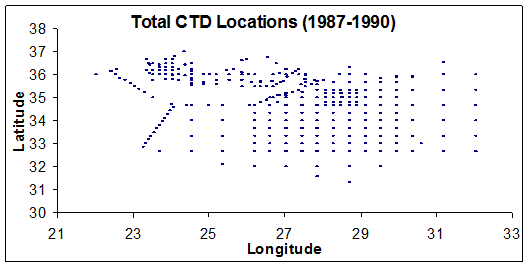Fig. 1. Locations of the 465 CTD (selected data profiles) stations in study area.

The available profiles were separated into two groups, 295 profiles contain the training data to be used for model fitting (Figure 2) and 170 profiles for independent verification (Figure 3). Figure 4, represents scatter plots of TS of the study area at different depths. The spread in salinity values at surface until 190 m depth is significant greater than the rest depths. The salinity profiles reveal some variability in the surface mixed layer commonly reaches nearly 1.00 psu. The mixed layer commonly reaches 30 meter or more, indicating that surface salinity is a useful indicator of near surface salinity in this region.

The mean and standard deviations of salinity and temperature profiles are representing in (Figure 5). Correlation coefficients between S(z) and T(z), are shown in (Figure 6). Correlation of salinity with temperature is low and negative value in the upper 70 m, nearly zero in 70 m, small and positive between about 70 m and 190 m, and the correlation increase below 190 m depth. The reversal sign of these correlations near 70 m reflects the presence of the salinity maximum (vertical displacements causing changes in salinity and temperature to have opposite signs). Correlation of salinity with surface salinity is high in the above 100m and decrease but still positive until 200 m depth, then under 200m inverse to negative sign until 425m depth.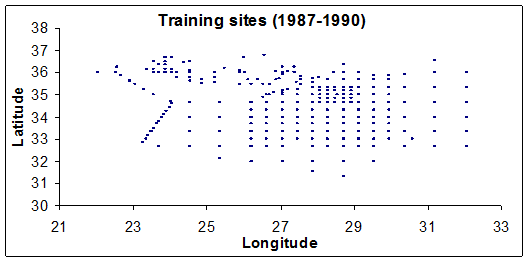Fig. 2. Locations of the 295 CTD training stations in the study area.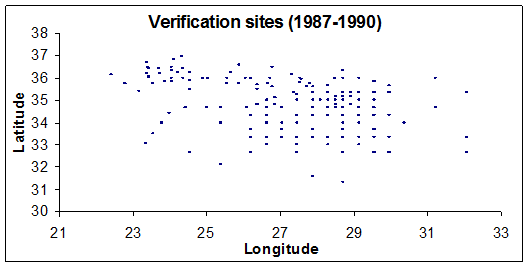Fig. 3. Locations of the 170 CTD verification stations in the study area.

2.2. Regression Methods (Models)

The strategy for estimating salinity is to identify regression models for each pressure level that explain the data in (Figure 4). The talent of these models is to be assessed against the verification data set for the corresponding pressure levels. The scatter plot (Figure 4) suggests that salinity might be modeled by polynomial of temperature of first (linearly) or higher degrees and that proved to be the case. Different degrees of Polynomial of temperature were fitted to the training data at each depth level. Eight of regression models were applies at each depth level, four of them corresponding to four combinations of temperature (linear, quadratic, cubic and 4th degree). The fifth regression model was combined between surface salinity and the 4th model for the upper 190m and only 4th model for the rest depths:

S = P1(T) = ao + a1T                               (1)

S = P2(T) = ao + a1T + a2T2                     (2)

S = P3(T) = ao + a1T + a2T2 + a3T3           (3)

S = P4(T) = ao + a1T + a2T2 + a3T3 + a4T4            (4)

S = P4(T) + S(0) = ao + a1T + a2T2 + a3T3 + a4T4+ a5S(0)  (5)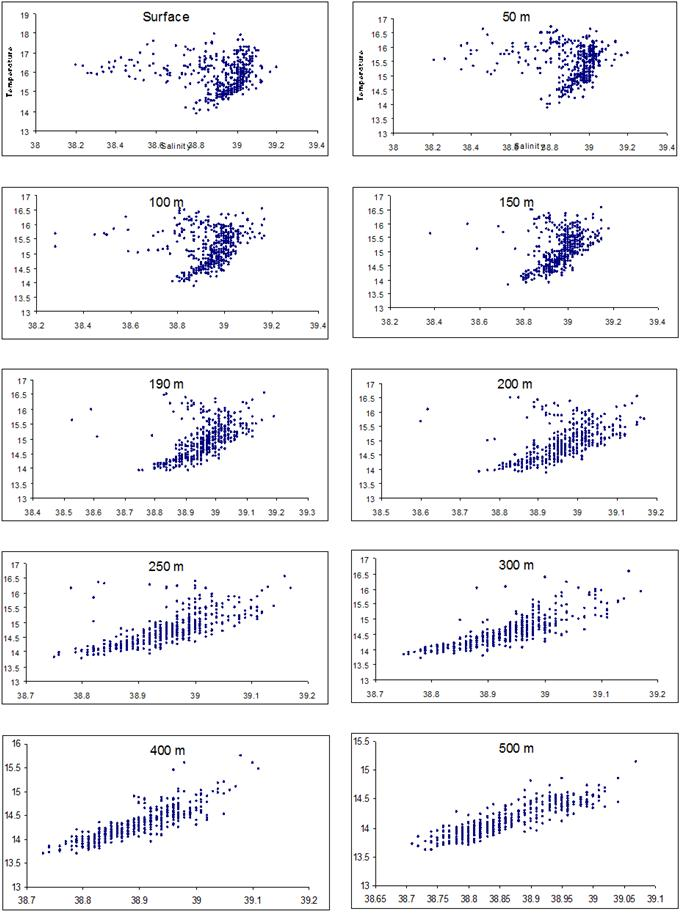Fig. 4. Scatter plots of Temperature and Salinity CTD data of the study area at different depths.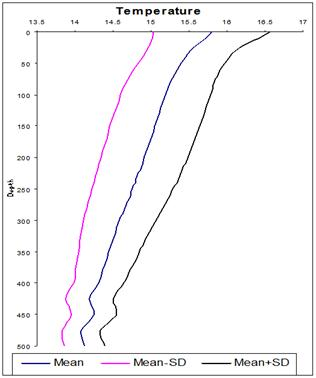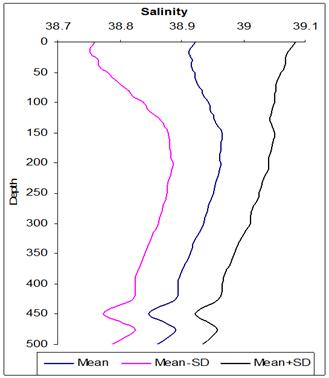Fig. 5. Mean values of temperature (left) and salinity (right) represent by blue curve for 465 data sets in this study, and standard deviations from mean by other curves.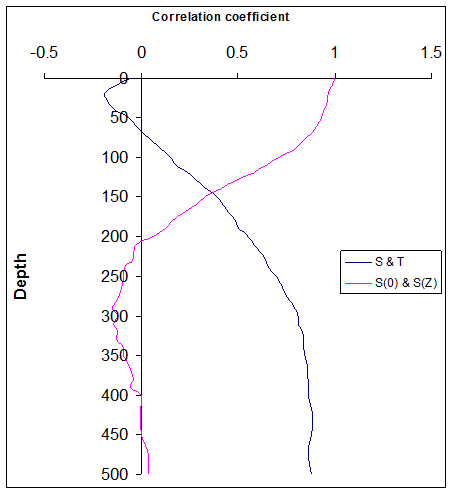Fig. 6. Blue curve represents correlation coefficient between salinity S(z) and temperature T(z) and magenta curve between S(0) and S(Z) at different depth interval.

The regression models number six, seven and eight also were used at each depth corresponding to the combinations of temperature (quadratic), Longitude and Latitude (model six) and day of the year (model seven). Model eight the same as model 7 with cubic temperature:

S = P2 (T) + x + y = ao + a1T + a2T2 + a3x + a4y                 (6)

S = P2 (T) + x + y + d = ao + a1T + a2T2 + a3x + a4y + a5d (7)

S = P3 (T) + x + y +d = ao + a1T + a2T2 + a3T3 + a4x + a5y + a6d (8)

Where, S denotes the estimate for salinity; T, S(0), d, x, y denote observed temperature, surface salinity, day of the year (Julian day), Longitude and Latitude (geographic location) respectively; and where the coefficients (a0, a1, a2, a3, a4, a5 and a6) were specified for the each model by fitting to the training data.

3. Results and Discussions

Salinity profiles Estimated from eight models

For the upper 500 m Salinity profiles were estimated by using eight regression models of different combination methods. These models apply for the 170 profiles of the verification profiles. Root mean square errors (RMSE) were computed between the estimated and measured salinities for the verification profiles for all models (Figure 7).

3.1. The Temperature Polynomial Models

For the first four types of models eq. (1 - 4) the RMSE decreased with depth. In the study area, for depth equal to/greater than 250 m, the high correlation between temperature and salinity (Figure 6) allows a 2nd, 3rd and 4th degrees of polynomial of temperature to estimate salinity with (RMSE) less than 0.05 psu (Figure 7). The RMSE was smaller than 0.03 psu at depth equal to/greater 400 m. Thacker and Sindlinger, (2007) , concluded that there is no empirical function of temperature can provide an accurate of salinity estimation near to the surface due to the TS relationship is less well defined. In this study, the RMSE value was fluctuated between 0.12 - 0.15 psu for the first 50 m depth (negative TS relationship), between 50 and 100m it was reached to 0.08 – 0.12 psu (with TS low positive relationship), between 100 – 200m the errors was decreased to 0.05 – 0.08 psu (with increasing TS relationship) and between 200 – 250m it was reached to 0.04 – 0.05 psu. The first model was less accurate to estimate the salinity for depths below 100m than the other three models.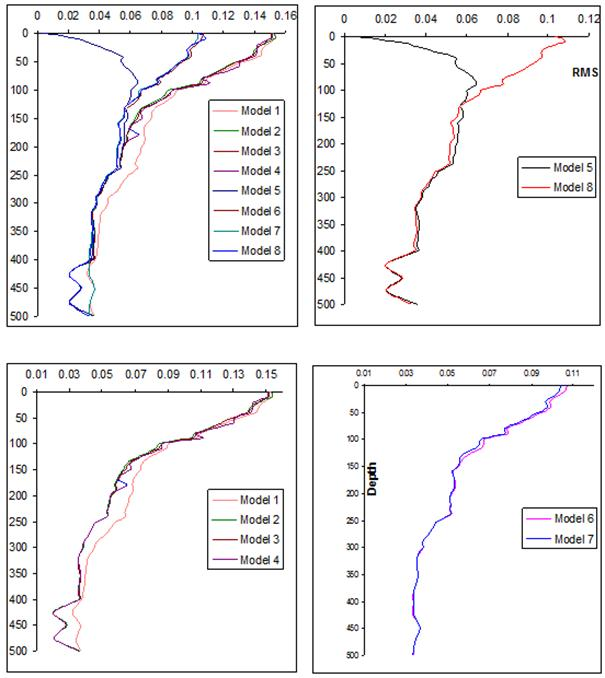Fig. 7. Root mean square error of estimating salinity profiles for verification data.

3.2. Temperature and Surface Salinity

The model number five eq. (5) suggested getting a benefit of high correlation between surface salinity S(0) and the salinity S(Z) for the upper 100 m depth (0.71 to 1) and decreased until 190 m depth. Indeed, the correlation between S(0) and S(Z) are dramatically decreased below 190m depth (Figure 6) and then inverse to negative sign, this situation encourage us to exclude surface salinity from the model five in the rest depths (i.e. 200-500m) and instead of that apply only 4th degree of polynomial of temperature for the rest depths (positive correlation of TS was increased from 0.55 to 0.88). Usually the surface salinity is using of to capture most of variability that characterizes the upper several tens of meters. The RMSE values for the first 50m depth was between 0.02 – 0.05 psu which was illustrates better than the output from temperature polynomial models, between 50 – 100m it was increased to 0.06 psu, between 100 – 200m decreased to 0.05 psu, and below 200m fluctuated between 0.02 – 0.05 psu. The RMSE range values at all depths between surface and 190m depth level were represented more accurate than previous temperature polynomial models. The RMSE range values for the rest depths were the same as output from 4th model (Figure 7).

All the models mentioned before are expected to do best results in application for homogenous water mass. However, for reliable and meaningful statistics, data must be drawn from the study area (10°´6°), over which water properties in the horizontal gradients may contribute significantly to the variances about the mean profiles. The following sections will test the possibility of capturing part of this variability, the Latitude y and Longitude x would be added to the other salinity predictors, because Mediterranean Sea climatologically structure is primarily zonal . In the following methods, the geographic location were added to the 2nd model as shown in eq. (6); also geographic location and day of the year added to the 2nd model as shown in eq. (7); again geographic location and day of the year added to the 3rd model as shown in eq. (8). These methods have been suggested to apply in case of absent of surface salinity and depend only on water temperature profiles measurement (as in Temperature polynomial models) in addition to geographic location and day of year.

3.3. Temperature, Longitude and Latitude

In comparison between the results of model number six eq. (6) and temperature polynomial models (equations 1 – 4), this model was enhanced RMSE (0.09-0.1) for depths until 50m, between 50 – 100m it was decreased to 0.067 psu, between 100 – 200m it was decreased to 0.051 psu, and between 200 - 400m depth RMSE values were decreased in the same trend as 2nd, 3rd and 4th models. RMSE values were slightly increased between 400-500m depth (Figure 7).

3.4. Temperature, Longitude, Latitude and Day of Year (Model 7)

The model number seven eq. (7) output results illustrate that RMSE value enhanced salinity estimation until 250 m depth than temperature polynomial methods (Figure 7). RMSE value at 30 m depth was 0.09 psu (model 7) while it was roughly 0.14 psu (models 1-4). There is no more information added by this model below 250m depth. RMSE values (Figure 7) for salinity estimation was decreased from surface water until 500m depth as the same trend of the previous method (model number 6) with little enhancement for the upper 130m.

3.5. Temperature, Longitude, Latitude and Day of Year (Model 8)

The output results of model (8) eq. (8) coincides with model (6) and (7) until 400m depth, and better than both models between 400 – 500m depth (Figure 7). Model (8) decreased RMSE until 240m depth than Models (1 – 4) and coincides with them for the rest depths, and also it decreased RMSE below 130m depth until 400m depth than model (5) and coincides with it between 400-500m depth.

The output results from the eight methods (models) of estimating salinity profiles have been presented in the present study. The curves had shown in (Figure 7) sort themselves into different classes. The first four models are nearly indistinguishable near 100 m depth. Near surface, the largest errors are associated with 2nd model. However model number 8 is more enhanced for upper 100m depth than the first 4 models. As expected when surface salinity added it will reduce the RMSE estimation. Surface salinity information addition to the 4th model reduces the RMSE to 0.05 psu in the upper 50 m.

Model (5) performs the best in upper 130m depths, while the model (8) performs the best for rest depths (i.e.; below 130m depth). To illustrate the ability of the model (5 & 8) to replicate individual salinity profiles, all 170 observed and estimated salinity profiles at each selected interval of the verification data set are displayed in (Figure 8). In addition, 32 samples of these observed and estimated salinity profiles are illustrated in (Figure 9); the temperature profiles of these samples are represented in (Figure 10).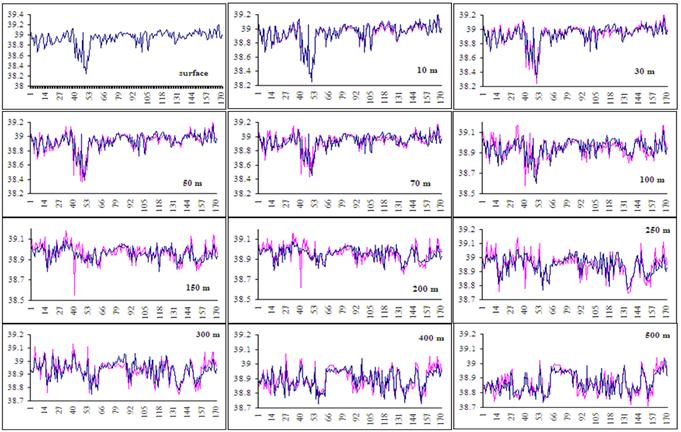Fig. 8. Measured (blue) and estimated (pink) salinity for verification data in some of selected data levels.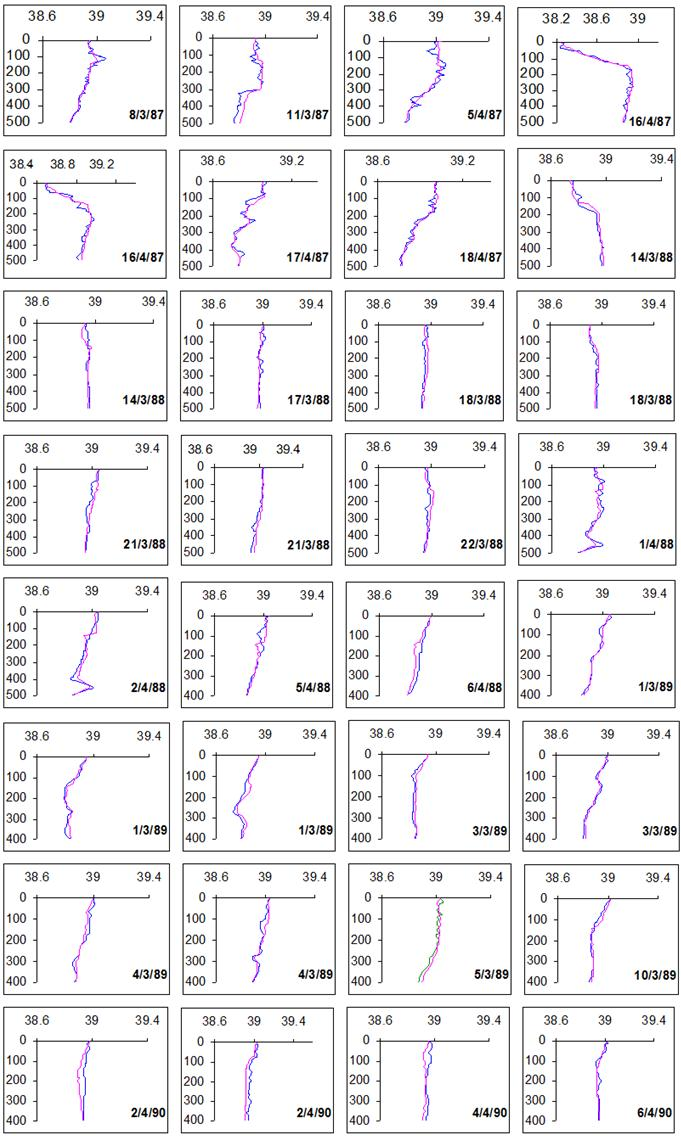Fig. 9. Estimation (pink) and observed (blue) salinity profiles for the 32 casts (this casts were not used in establishing statistical relationships).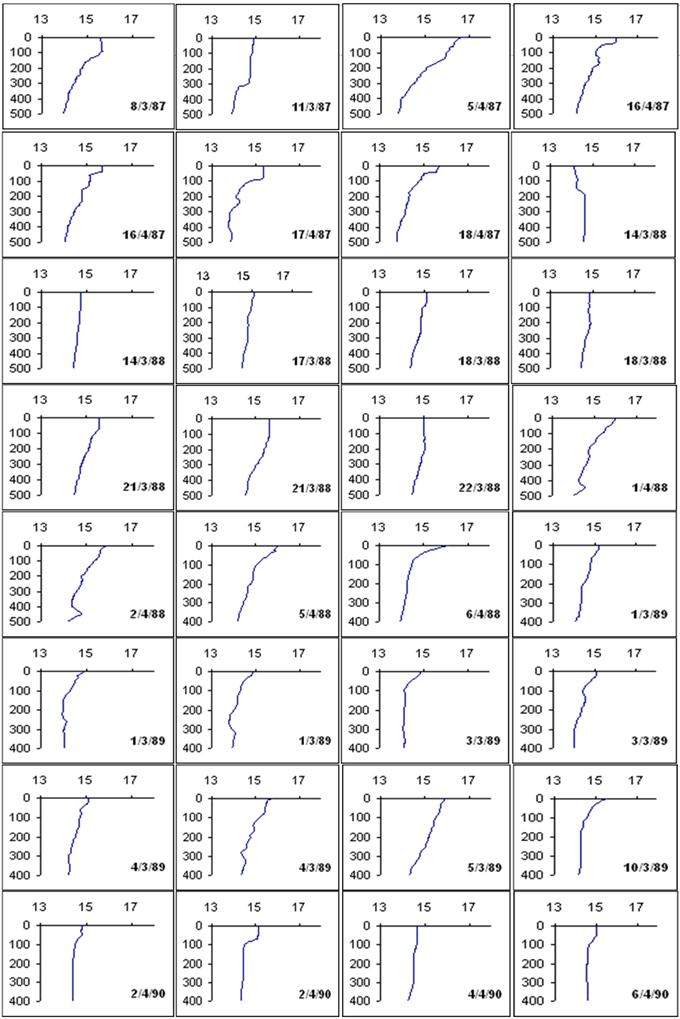Fig. 10. Observed temperature profiles that were used in estimating the salinity profiles in Fig. 9.

4. Summary and Conclusion

Only 465 temperature and salinity profiles were selected for this study, has been taken from research vessels Yakov Gakkov and Vladimir Parshin, of former Soviet Union during spring months of the period 1987-1990. The profiles were separated into two groups, 295 profiles containing the training data were used for model fitting and 170 profiles for independent verification. Eight methods (models) were used for estimating salinity profiles in the southeastern Mediterranean Sea. The local regression approach has been shown to be suitable for the Southeastern Mediterranean Sea between 22°E to 32°E and 31°N to 37°N to characterizing the spatially varying T-S relationship. While the results found here might not apply everywhere. Surface salinity added to 4th model to build model 5, it is interesting to note it might allow RMSE for estimates salinity at 30m depth reduced to 0.04 psu. Model 6 was sufficient to capture T-S co-variability, and both Longitude and Latitude provide helpful information about salinity, even at 500 m depth, as did when day of year has been added (model 7). RMSE decreased for estimates salinity at depths between 400-500m when second-degree polynomial of temperature replaced by third-degree polynomial of temperature (model 8). The combination between the fifth (performs the best in upper 130m depths) and eighth models (performs the best for rest depths below 130m depth) gives the best results to estimate the salinity profiles.

References

1. F. Reseghetti, "Empirical reconstruction of salinity from temperature profiles with phenomenological constrains". Ocean Science Discussion, (2007), (4), 1-39.
2. W.C Thacker, "Estimating salinity to complement observed temperature: 1. Gulf of Mexico". Journal of Marine Systems, (2007), (65), 224-248.
3. H. Stommel, "Note on the use of the T–S correlation for dynamic height anomaly calculations". Journal of Marine Research, (1947), (6), 85–92.
4. W.C Thacker, "Estimating Salinity between 25° and 45° in the Atlantic Ocean using Local Regression". Journal of Atmospheric and Oceanic Technology, (2008), (25), 114-130.
5. G.R. Flierl, "Correcting expendable bathythermograph (XBT) data for salinity effects to compute dynamic heights in Gulf Stream rings". Deep-Sea Research, (1978), (25), 129–134.
6. J.R. Donguy, G. Eldin, and K. Wyrtki, "Sea level and dynamic topography in the western Pacific during 1982–1983 El Nino". Tropical Ocean-Atmosphere Newsletter, (1986), (36), 1–3.
7. W.S. Kessler, and B.A. Taft, "Dynamic heights and zonal geostrophic transports in the central tropical Pacific during 1979 –1984". Journal of Physical Oceanography,(1987), (17), 97–122.
8. A. Troccoli, and K. Haines, "Use of the temperature – salinity relation in a data assimilation context". Journal of Atmospheric and Oceanic Technology, (1999), (16), 2011–2025.
9. F.C. Vossepoel, R.W. Reynolds, and L. Miller, "Use of sea level observations to estimate salinity variability in the tropical Pacific". Journal of Atmospheric and Oceanic Technology, (1999), (16), 1401–1415.
10. D.V. Hansen, and W.C. Thacker, "Estimation of salinity profiles in the upper ocean". Journal of Geophysical Research,(1999), (104), 7921–7933.
11. M.A.A. Hussein, M.A. Said, and A.A. Radwan, "Estimation of salinity profiles in the southeastern Mediterranean off the Egyptian coast".Journal of King Abdul-Aziz University (JKAU): Marine Science, (2011), (22), 79-95.
12. D.N. Fox, C.N. Barron, M. R. Carnes, M. Booda, G. Peggion, and J.V. Gurley, " The modular ocean data assimilation system". Oceanography, (2002), 15(1), 22–28.
13. W.C. Thacker, and L. Sindlinger, "Estimating salinity to complement observed temperature: 2. Northwestern Atlantic". Journal of Marine Systems, (2007), (65), 249-267.
14. K.T. Hjelmervik, and K. Hjelmervik, "Estimating temperature and salinity profiles using empirical orthogonal functions and clustering on historical measurements". Ocean Dynamics, (2013), (63), 809-821.
15. M.B. Gueye, A. Niang, S. Arnault, S. Thiria, and M. Crépon, "Neural approach to inverting complex system: Application to ocean salinity profile estimation from surface parameters".Computers & Geosciences, (2014), (72), 201-209.
16. CIESM website http://www.ciesm.org/marine/campaigns/sovietcruises.htm

 Contents 1. 2. 2.1. 2.2. 3. 3.1. 3.2. 3.3. 3.4. 3.5. 4.
Article ToolsAbstractPDF(4110K)